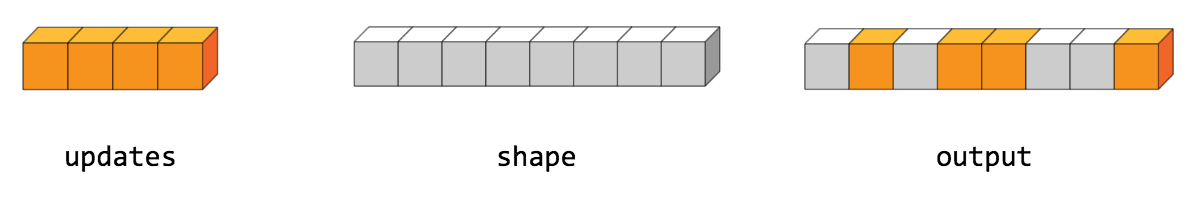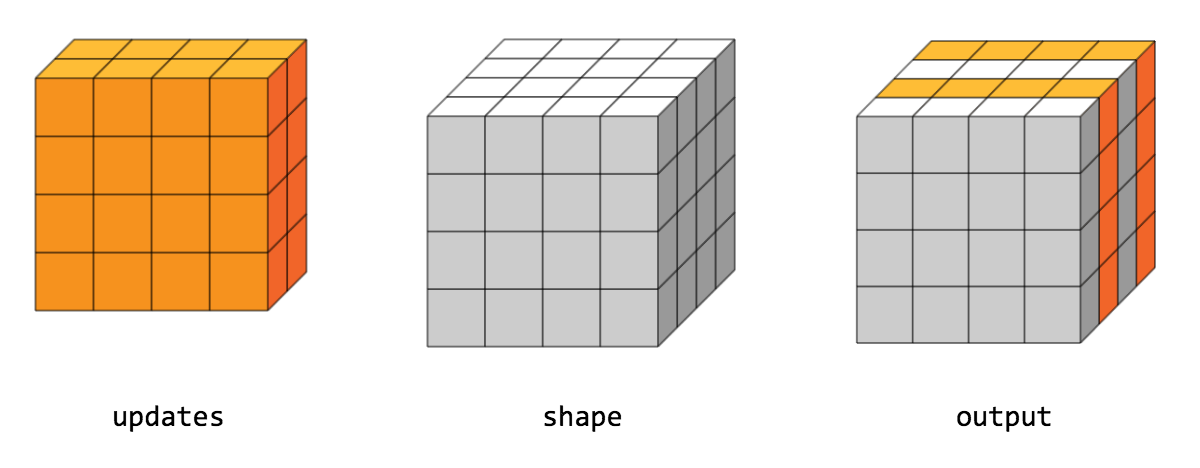# tf.scatter_nd

Defined in generated file: `python/ops/gen_array_ops.py`

Scatter `updates` into a new tensor according to `indices`.

### Aliases:

• `tf.compat.v1.manip.scatter_nd`
• `tf.compat.v1.scatter_nd`
• `tf.compat.v2.scatter_nd`
``````tf.scatter_nd(
indices,
shape,
name=None
)
``````

Creates a new tensor by applying sparse `updates` to individual values or slices within a tensor (initially zero for numeric, empty for string) of the given `shape` according to indices. This operator is the inverse of the `tf.gather_nd` operator which extracts values or slices from a given tensor.

This operation is similar to tensor_scatter_add, except that the tensor is zero-initialized. Calling `tf.scatter_nd(indices, values, shape)` is identical to `tensor_scatter_add(tf.zeros(shape, values.dtype), indices, values)`

If `indices` contains duplicates, then their updates are accumulated (summed).

WARNING: The order in which updates are applied is nondeterministic, so the output will be nondeterministic if `indices` contains duplicates -- because of some numerical approximation issues, numbers summed in different order may yield different results.

`indices` is an integer tensor containing indices into a new tensor of shape `shape`. The last dimension of `indices` can be at most the rank of `shape`:

``````indices.shape[-1] <= shape.rank
``````

The last dimension of `indices` corresponds to indices into elements (if `indices.shape[-1] = shape.rank`) or slices (if `indices.shape[-1] < shape.rank`) along dimension `indices.shape[-1]` of `shape`. `updates` is a tensor with shape

``````indices.shape[:-1] + shape[indices.shape[-1]:]
``````

The simplest form of scatter is to insert individual elements in a tensor by index. For example, say we want to insert 4 scattered elements in a rank-1 tensor with 8 elements.In Python, this scatter operation would look like this:

``````    indices = tf.constant([, , , ])
updates = tf.constant([9, 10, 11, 12])
shape = tf.constant()
with tf.Session() as sess:
print(sess.run(scatter))
``````

The resulting tensor would look like this:

``````[0, 11, 0, 10, 9, 0, 0, 12]
``````

We can also, insert entire slices of a higher rank tensor all at once. For example, if we wanted to insert two slices in the first dimension of a rank-3 tensor with two matrices of new values.In Python, this scatter operation would look like this:

``````    indices = tf.constant([, ])
updates = tf.constant([[[5, 5, 5, 5], [6, 6, 6, 6],
[7, 7, 7, 7], [8, 8, 8, 8]],
[[5, 5, 5, 5], [6, 6, 6, 6],
[7, 7, 7, 7], [8, 8, 8, 8]]])
shape = tf.constant([4, 4, 4])
with tf.Session() as sess:
print(sess.run(scatter))
``````

The resulting tensor would look like this:

``````[[[5, 5, 5, 5], [6, 6, 6, 6], [7, 7, 7, 7], [8, 8, 8, 8]],
[[0, 0, 0, 0], [0, 0, 0, 0], [0, 0, 0, 0], [0, 0, 0, 0]],
[[5, 5, 5, 5], [6, 6, 6, 6], [7, 7, 7, 7], [8, 8, 8, 8]],
[[0, 0, 0, 0], [0, 0, 0, 0], [0, 0, 0, 0], [0, 0, 0, 0]]]
``````

Note that on CPU, if an out of bound index is found, an error is returned. On GPU, if an out of bound index is found, the index is ignored.

#### Args:

• `indices`: A `Tensor`. Must be one of the following types: `int32`, `int64`. Index tensor.
• `updates`: A `Tensor`. Updates to scatter into output.
• `shape`: A `Tensor`. Must have the same type as `indices`. 1-D. The shape of the resulting tensor.
• `name`: A name for the operation (optional).

#### Returns:

A `Tensor`. Has the same type as `updates`.